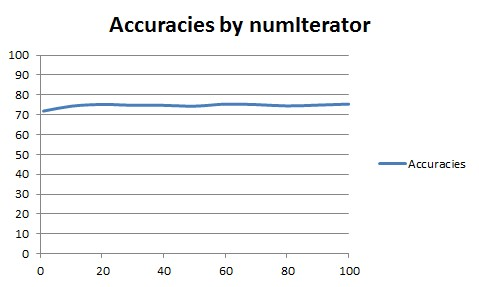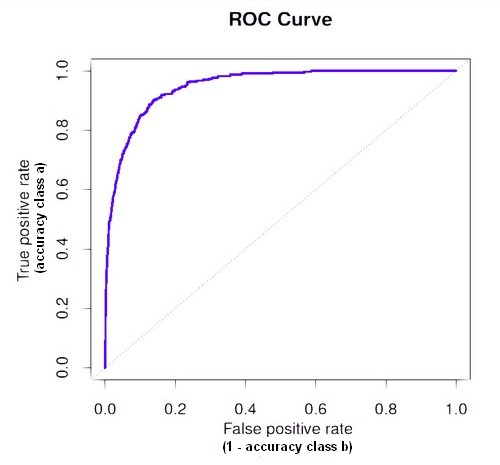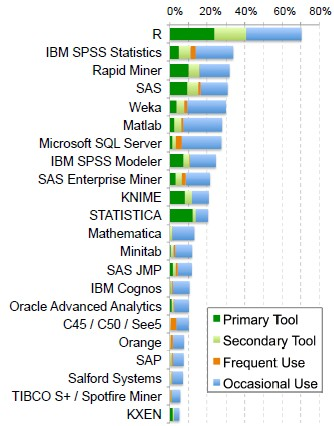# Machine Learning - (C4.5|J48) algorithm

C4.5 algorithm is a classification algorithm producing decision tree based on information theory

C4.5 is from Ross Quinlan (known in Weka as J48 J for Java). He fixes ID3 to the C4.5 algorithm in 1993.

The best attribute to split on is the attribute with the greatest information gain.

## Documentation / Reference

Discover MoreData Mining - (Boosting|Gradient Boosting|Boosting trees)

Boosting forces new classifiers to focus on the errors produced by earlier ones. boosting works by aggressively reducing the training error Gradient Boosting is an algorithm based on an ensemble of decision...Data Mining - Decision Tree (DT) Algorithm

Desicion Tree (DT) are supervised Classification algorithms. They are: easy to interpret (due to the tree structure) a boolean function (If each decision is binary ie false or true) Decision trees...Data Mining - Decision boundary Visualization

Classifiers create boundaries in instance space. Different classifiers have different biases. You can explore them by visualizing the classification boundaries. Logistic Regression method produces...Statistics - (Confidence|likelihood) (Prediction probabilities|Probability classification)

Prediction probabilities are also known as: confidence (How confident can I be of this prediction?). or likelihood: (How likely is this prediction to be true?) They gives the probability of a predicted...Statistics - ROC Plot and Area under the curve (AUC)

The Area Under Curve (AUC) metric measures the performance of a binary classification. In a regression classification for a two-class problem using a probability algorithm, you will capture the probability...Weka

is an open-source project in machine learning, Data Mining. is a comprehensive collection of machine-learning algorithms for data mining tasks written in Java....